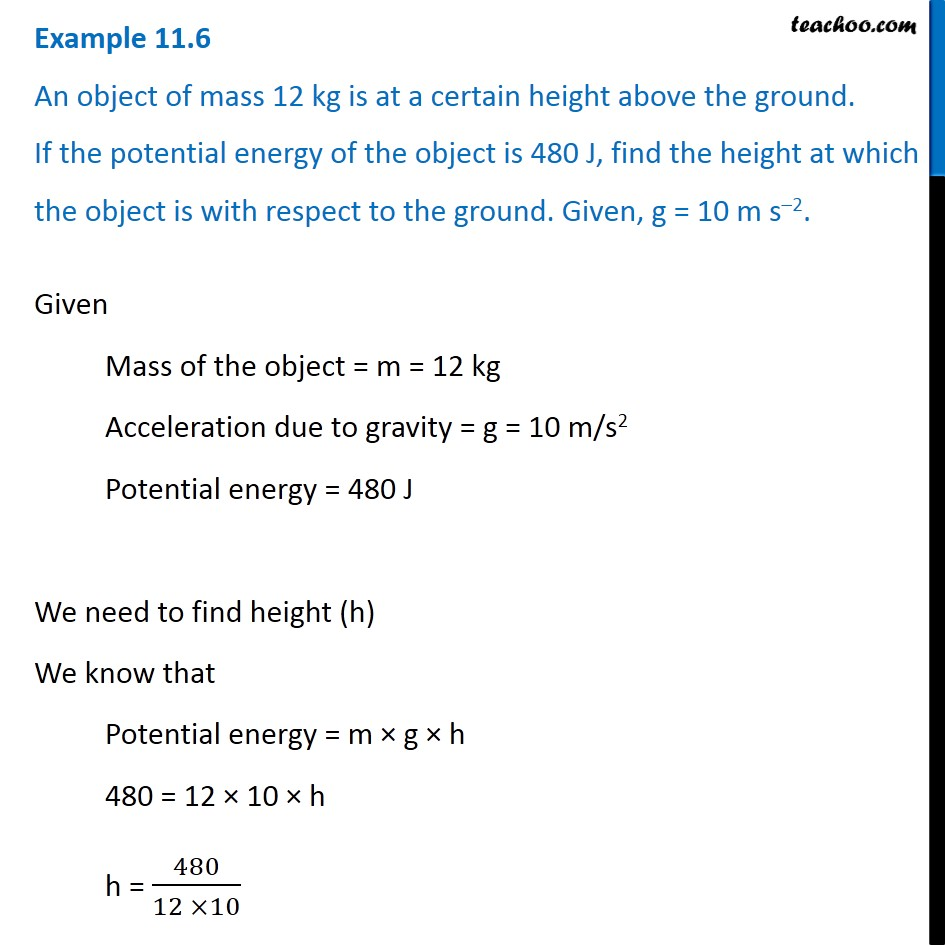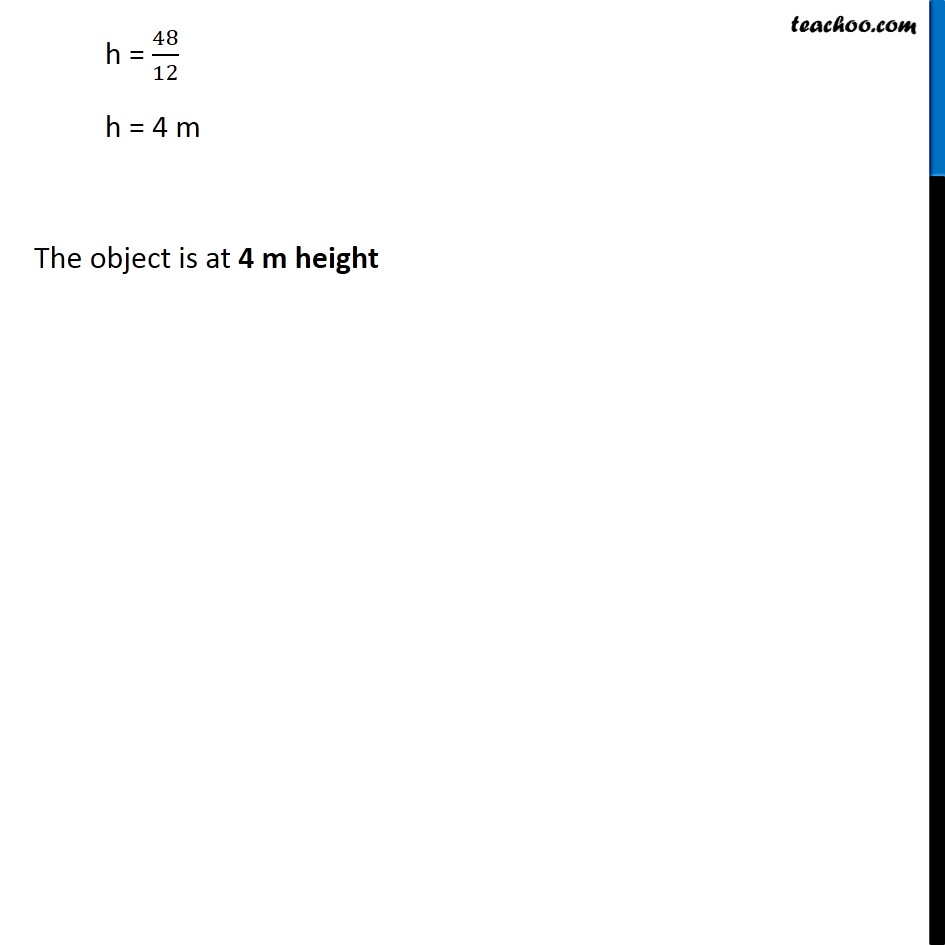Examples from NCERT Book

Class 9
Chapter 11 Class 9 - Work and EnergyLearn in your speed, with individual attention - Teachoo Maths 1-on-1 Class

### Transcript

Example 11.6 An object of mass 12 kg is at a certain height above the ground. If the potential energy of the object is 480 J, find the height at which the object is with respect to the ground. Given, g = 10 m s–2. Given Mass of the object = m = 12 kg Acceleration due to gravity = g = 10 m/s2 Potential energy = 480 J We need to find height (h) We know that Potential energy = m × g × h 480 = 12 × 10 × h h = 480/(12 ×10) h = 48/12 h = 4 m The object is at 4 m height